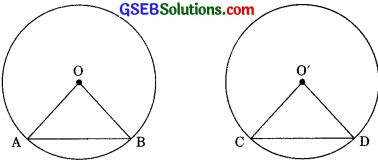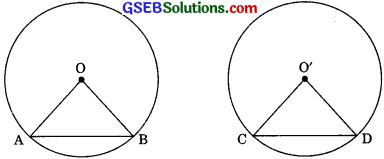# GSEB Solutions Class 9 Maths Chapter 10 Circles Ex 10.2

Gujarat Board GSEB Solutions Class 9 Maths Chapter 10 Circles Ex 10.2 Textbook Questions and Answers.

## Gujarat Board Textbook Solutions Class 9 Maths Chapter 10 Circles Ex 10.2

Question 1.
Recall that two circles are congruent if they have the same radii. Prove that equal chords of congruent circles subtend equal angles at their centres.
Solution:
Given: AB and CD are two equal chords of congruent circles with centres O and O’ respectively.
To Prove: ∠AOB = ∠CO’D.
Proof: In ΔOAB and ΔO’CD,
OA = O’C [Radii of congruent circles]
OB = O’D [Radii of congruent circles]AB = CD [Given]
ΔOAB = ΔO’CD [SSS Rule]
∠AOB = ∠CO’D. [CPCT]Question 2.
Prove that if chords of congruent circles subtend equal angles at their centres, then the chords are equal.
Solution:
Given: ∠AOB and ∠CO’D are the two equal angles subtended by the chords AB and CD of two congruent circles with centres O and O’ respectively.
To Prove: AB = CDProof: In ΔOAB and ΔO’CD
OA = O’C [Radii of congruent circles]
OB = O’D [Radii of congruent circles]
∠AOB = ∠CO’D [Given]
∴ ΔOAB = ΔO’CD [SAS Rule]
∴ AB = CD [CPCT]